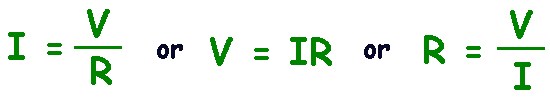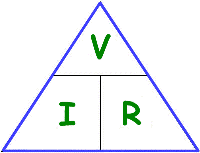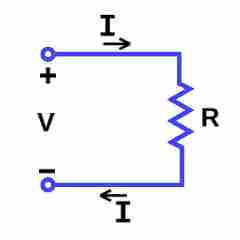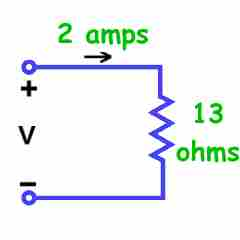Parents and Teachers: Support Ducksters by following us onor.

Science >> Physics for Kids

# Physics for Kids

## Ohm's Law

One of the most important and basic laws of electrical circuits is Ohm's law which states that the current passing through a conductor is proportional to the voltage over the resistance.

Equation

Ohm's law may sound a bit confusing when written in words, but it can be described by the simple formula:where I = current in amps, V = voltage in volts, and R = resistance in ohms

This same formula can be also be written in order to calculate for the voltage or the resistance:Triangle

If you ever need help in remembering the different equations for Ohm's law and solving for each variable (V, I, R) you can use the triangle below.As you can see from the triangle and the equations above, voltage equals I times R, current (I) equals V over R, and resistance equals V over I.

Circuit Diagram

Here is a diagram showing I, V, and R in a circuit. Any one of these can be calculated using Ohm's law if you know the values of the other two.How Ohm's Law Works

Ohm's law describes the way current flows through a resistance when a different electric potential (voltage) is applied at each end of the resistance. One way to think of this is as water flowing through a pipe. The voltage is the water pressure, the current is the amount of water flowing through the pipe, and the resistance is the size of the pipe. More water will flow through the pipe (current) the more pressure is applied (voltage) and the bigger the pipe is (lower the resistance).

Example Problems

1. If the resistance of an electrical circuit is increased, what will happen to the current assuming the voltage remains the same?

Answer: The current will decrease.

2. If the voltage across a resistance is doubled, what will happen to the current?

Answer: The current will double as well.

Explanation: If you look at the equation V= IR, if R stays the same then if you multiple V*2 (double the voltage), you must also double the current for the equation to remain true.

3. What is the voltage V in the circuit shown?Answer: V = I * R = 2 x 13 = 26 volts

Interesting Facts about Ohm's Law
• It is generally applied only to direct current (DC) circuits, not alternating current (AC) circuits. In AC circuits, because the current is constantly changing, other factors such as capacitance and inductance must be taken into account.
• The concept behind Ohm's law was first explained by German Physicist Georg Ohm who the law is also named after.
• The tool for measuring volts in an electric circuit is called a voltmeter. An ohmmeter is used for measuring resistance. A multimeter can measure several functions including voltage, current, resistance, and temperature.
Activities

More Electricity Subjects

 Circuits and Components Intro to Electricity Electric Circuits Electric Current Ohm's Law Resistors, Capacitors, and Inductors Resistors in Series and Parallel Conductors and Insulators Digital Electronics Other Electricity Electricity Basics Electronic Communications Uses of Electricity Electricity in Nature Static Electricity Magnetism Electric Motors Glossary of Electricity Terms

Science >> Physics for KidsFollow us onor